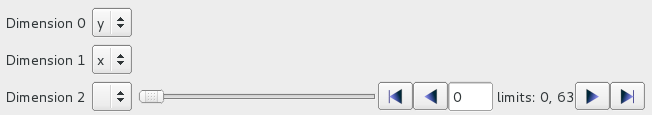# NumpyAxesSelector:Widget to select a view from a numpy array¶

This module defines a widget able to convert a numpy array from n-dimensions to a numpy array with less dimensions.

class NumpyAxesSelector(parent=None)[source]

Widget to select a view from a numpy array.The widget is set with an input data using setData(), and a requested output dimension using setAxisNames().

Widgets are provided to selected expected input axis, and a slice on the non-selected axis.

The final selected array can be reached using the getter selectedData(), and the event selectionChanged.

If the input data is a HDF5 Dataset, the selected output data will be a new numpy array.

dataChanged

Emitted when the input data change

selectedAxisChanged

Emitted when the selected axis change

selectionChanged

Emitted when the selected data change

customAxisChanged

Emitted when a custom axis change

clear()[source]

Clear the widget.

setAxisNames(axesNames)[source]

Set the axis names of the output selected data.

Axis names are defined from slower to faster axis.

The size of the list will constrain the dimension of the resulting array.

Parameters: axesNames (List[str]) – List of distinct strings identifying axis names
setCustomAxis(axesNames)[source]

Set the available list of named axis which can be set to a value.

Parameters: axesNames (List[str]) – List of customable axis names
setData(data)[source]

Set the input data unsed by the widget.

Parameters: data (numpy.ndarray) – The input data
data()[source]

Returns the input data.

Return type: Union[numpy.ndarray,None]
selectedData()[source]

Returns the output data.

This is equivalent to:

numpy.transpose(self.data()[self.selection()], self.permutation())

Return type: Union[numpy.ndarray,None]
permutation()[source]

Returns the axes permutation to convert data subset to selected data.

If permutation cannot be computer, it returns None.

Return type: Union[List[int],None]
selection()[source]

Returns the selection tuple used to slice the data.

Return type: tuple
setSelection(selection, permutation=None)[source]

Set the selection along each dimension.

tuple returned by selection() can be provided as input, provided that it is for the same the number of axes and the same number of dimensions of the data.

Parameters: selection (List[Union[int,slice,None]]) – The selection tuple with as one element for each dimension of the data. If an element is None, then the whole dimension is selected. permutation (Union[List[int],None]) – The data axes indices to transpose. If not given, no permutation is applied ValueError – When the selection does not match current data shape and number of axes.
setNamedAxesSelectorVisibility(visible)[source]

Show or hide the combo-boxes allowing to map the plot axes to the data dimension.

Parameters: visible – Boolean